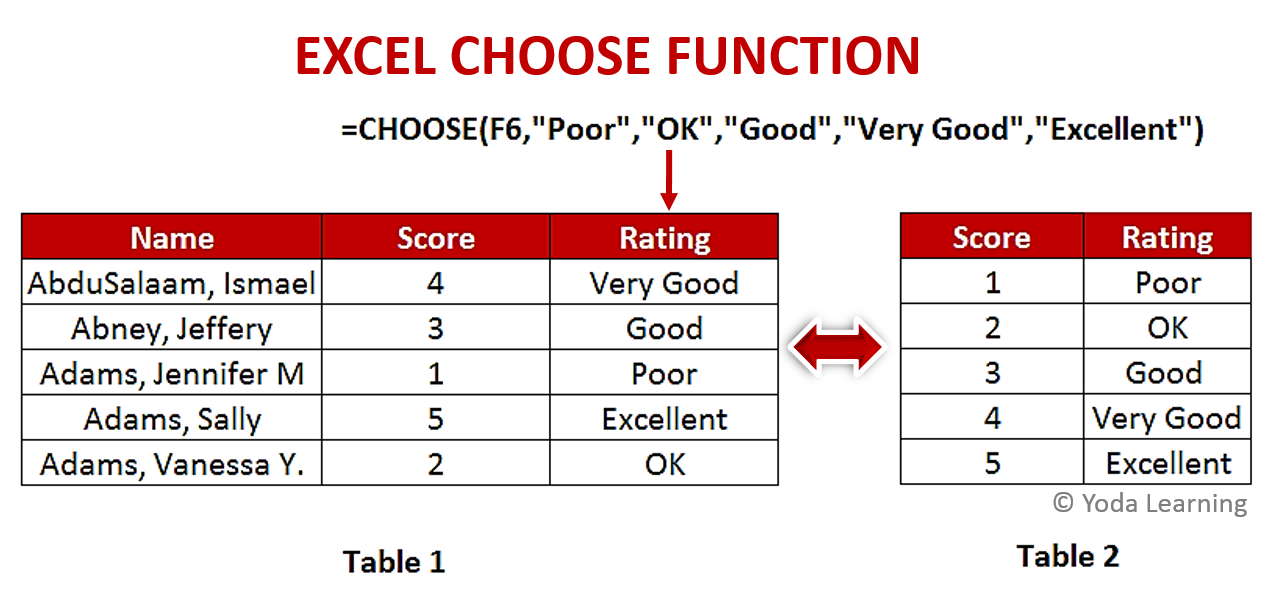What is the Syntax of Excel Choose Function? - KING OF EXCEL

## Saturday, December 14, 2019

### What is the Syntax of Excel Choose Function?

The syntax for writing CHOOSE Function is-
=CHOOSE (index_num, value1, [value2],…)
Where,
Index_num – The value to choose between 1 and 254
Value1 – The first value from which to choose
Value2 – The second value from which to choose
➡ Example:
There are many cases explained below where you can use the CHOOSE Function. Let’s take a simple example to understand the importance of this function.
As shown in picture below, we have two data tables, Table 1 & Table 2. The Table 1 consists of the name of candidates whose rating needs to be set depending upon their score. Table 2 has the rating with the score. Rating column in Table 1 can be filled by using VLOOKUP. But, one there is also a more easy way than VLLOKUP. Write a formula using CHOOSE Function and give the reference number first followed by the rating in sequence as given below.=CHOOSE(F6,”Poor”,”OK”,”Good”,”Very Good”,”Excellent”)

### ➡ Where Choose Function Can be used?

Applications of CHOOSE Function are as follows-
• Add an ordinal suffix to a number you can use a formula based on the CHOOSE Function to assign the suffix
• To create a custom weekday abbreviation, you can use a formula based on the CHOOSE Function and WEEKDAY Function
• Adding anything random to groups, use the RANDBETWEEN function along with the CHOOSE Function
• To map a date to any values you want use the CHOOSE Function
• To quickly fill a range of cells with random text values, you can use the CHOOSE and RANDBETWEEN functions

### ➡ Top 3 Points to Remember:

• You can choose the Index number between 1 and 254
• If you choose a negative or zero value as the index number, you will get a VALUE# error
• If the index number is a fraction, it is truncated to the lowest integer before being used

### ➡ Conclusion:

Here, we come to know about the CHOOSE Function. We have seen its purpose, uses, the formula to be used and where can we find this function. Hope your concept regarding the CHOOSE Function is clear and you can now carry out this function easily.
📤You download App EVBA.info installed directly on the latest phone here : https://www.evba.info/p/app-evbainfo-setting-for-your-phone.html?m=1